# GMAT Math : Calculating profit

## Example Questions

2 Next →

### Example Question #11 : Calculating Profit

The German Club wants to make and sell cookies in order to raise $500 for a field trip. The equipment they want to use costs$400 to rent and to operate, and the ingredients for the cookies cost 45 cents per cookie. The German Club wants to sell the cookies for $2.25 each. At this price, how many cookies will they need to sell in order to earn back the money they paid for the ingredients and the equipment rental and make a profit of$500?

Ifis the number of cookies sold, then which of the following equations represents the revenue function?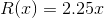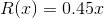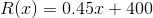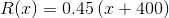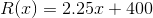Explanation:

The money raised from each cookie sold will be $2.25, so this is to be multilplied by number of cookiesto obtain the revenue in terms of. Therefore, the revenue function is. ### Example Question #12 : Calculating Profit Read the problem below: The Russian Club wants to make and sell cookies in order to raise$800 for a field trip. The equipment they want to use costs $350 to rent and to operate, and the ingredients for the cookies cost 35 cents per cookie. The Russian Club wants to sell the cookies for$1.75 each. At this price, how many cookies will they need to sell in order to earn back the money they paid for the ingredients and the equipment rental and make a profit of $800? Ifis the number of cookies sold, then which of the following inequalities can be solved to obtain an answer to this question? Possible Answers: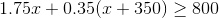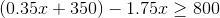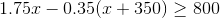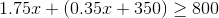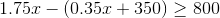Correct answer:Explanation: Letbe the number of cookies sold. The Russian Club will need to spend 35 cents, or$0.35, per cookie for the ingredients, plus $350 flat for the ingredients, so the cost function will be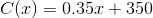They will sell the cookies for$1.75 each, thereby earning a revenue of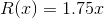They want to earn at least $800, so the profit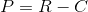must be at least this - or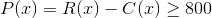Replacing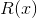and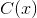with their definitions, the inequality becomes### Example Question #13 : Calculating Profit The Spanish Club wants to make and sell cookies in order to raise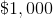for a field trip. The equipment they want to use costs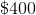to rent and to operate, and the ingredients for the cookies costcents per cookie. The Spanish Club wants to sell the cookies for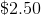each. At this price, how many cookies will they need to sell in order to earn back the money they paid for the ingredients and the equipment rental and make a profit of? Possible Answers: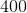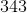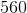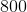Correct answer:Explanation: Letbe the number of cookies sold. The Spanish Club will need to spend 75 cents, or$0.75, per cookie for the ingredients, plus $400 flat for the ingredients, so the cost function will be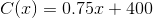They will sell the cookies for$2.50 each, thereby earning a revenue of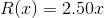They want to earn at least $1,000, so the profit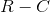must be at least this - or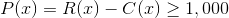Solve the inequality: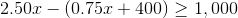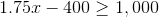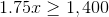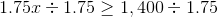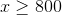The Spanish Club will need to sell 800 cookies. ### Example Question #14 : Calculating Profit Adam buys 12 broken cell phones for$50 each. If he fixes the cell phones and sells them each for $80, what is his total profit? Possible Answers:$260

$800$600

$960$360

$360 Explanation: Adam first buys 12 cell phones for$50 each, which means he spends:

12($50) =$600

He then sells the phones for $80 each, which means he earns: 12($80) = $960 The profit is the earnings minus the expenses, so Adam's profit is:$960 - $600 =$360

### Example Question #15 : Calculating Profit

It costs a company an average of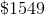to build one of their custom woodchippers. The company makes an average profit of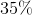of the cost of building a machine. To the nearest dollar, what is the average net profit the company makes per unit sold?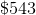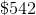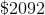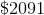Explanation:

The company makes an average net profit that isof the cost of building a machine. To calculate the average net profit in this case, simply do the following: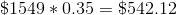So, to the nearest dollar, the company's net profit is.

Note: avoid the trap answer. Our unrounded answer is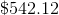, so we need to round down toinstead of rounding up to.

### Example Question #16 : Calculating Profit

The stock we just bought soaredpercent, in other words our position increased by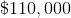. How much did we initially invest?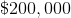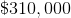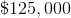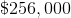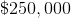Explanation:

A 55% increased of the unkown valueresulting as a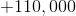increase can be written as follow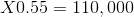.

So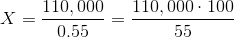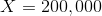, which is the initial value.

### Example Question #17 : Calculating Profit

Mary, a very respected day trader, has the following postions: She invested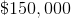in stock A,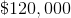in stock B and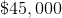in stock C. What is her total return, in dollars on this portfolio considering the fact that since she invested in these stocks, A went up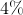, B went down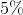and C increased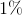.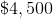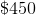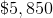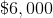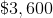Explanation:

To solve this problem, we simply have to multiply each position by their respective rate.

Note that we use a negative rate if the value decreased.

The final answer is then given by following equation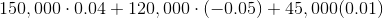and we end up with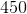.

### Example Question #18 : Calculating Profit

We have just lostpercent, or, of our investment, what was the amount invested before the loss?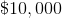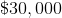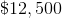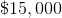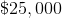Explanation:

To find the amount invested prior to the loss set up a proportion that represents what is given mathematically.

To write 60 percent of something is the same as,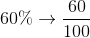If this is equivalent to \$6,000 then the statement to solve would read,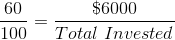.

From here cross multiply and divide to solve for the amount invested prior to the loss.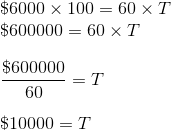2 Next →

Tired of practice problems?

Try live online GMAT prep today.